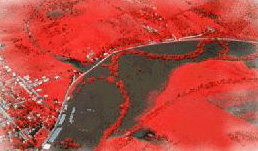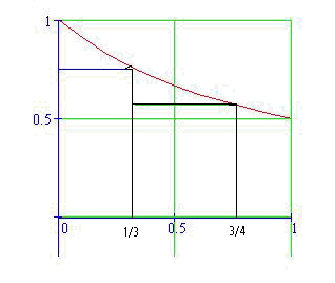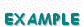Calculus on Demand at Dartmouth College Lecture 25 | Index | Lecture 27 Lecture 26## Resources

Math 3 Course Syllabus
Practice Exams

# Contents

In this lecture we consider a real-world application of modeling with accumulations by developing a case study on river flooding. This material is the extended application and culmination of our work in lectures 21-26.

### Quick Question

What is the approximate area under the graph of the function 1/(1 + x) on the interval [0,1] using the two inscribed rectangles on the intervals [0,1/3] and [1/3,3/4]? (You need not simplify your answer.)### Outline

Outline for Case Study: Flood Watch

### Textbook

Case Study: Flood Watch

### Quiz

Case Study: Flood Watch Quiz

### Examples

•Calculate the centroid of the region between the curves f(x) = x2 + 2 and g(x) = 2x + 5 for x in the interval [–1, 3].
•A certain river has rainfall discharge data and rainfall recorded during and after a storm. Find the base flow of the river, the total volume of rainfall discharge, and the lag time between the rainfall event and the rainfall discharge.
•A flower grows at varying rates, measured at the beginning of each month and halfway through each month, according to a chart. What is the approximate height of the flower after three months? Consider a second flower that requires fertilizer to grow, and compute the lag time between the application of fertilizer and the flower's growth.

### Videos

Lecture 25 | Index | Lecture 27